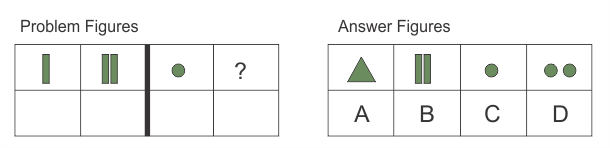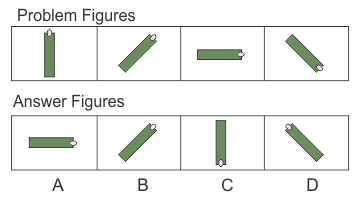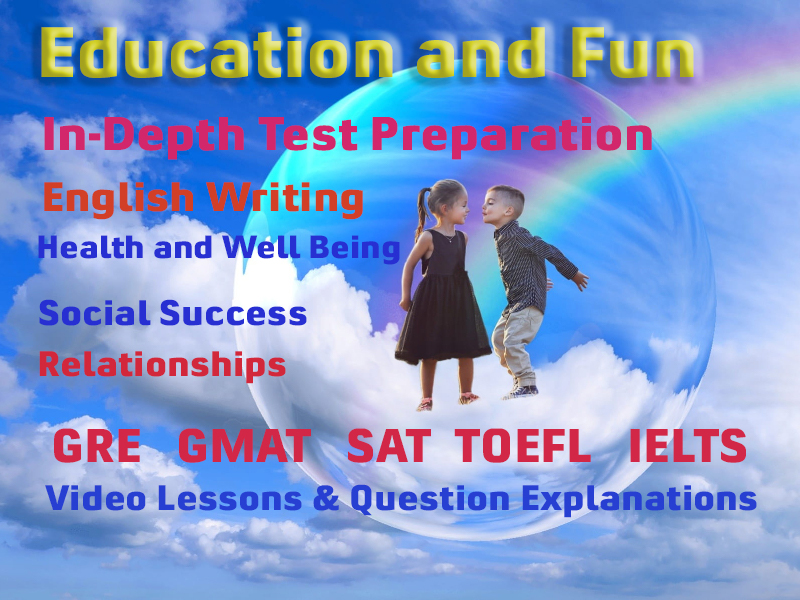# Intelligence Matrices - Picture Pattern Questions

Most of the intelligence tests rely up to a great extent on language proficiency and previous knowledge of the candidate. To neutralize the effect of social, environment, culture, and schooling institutions and at the same time ensure equal measurement on one scale, non-verbal tasks called matrices are used. These question require no language proficiency to solve.

Non-verbal matrix questions go under three broad categories:

• Analogies
• Classification
• Series

### Picture Pattern - Analogies

In this type of questions figures are divided into two parts - Problem figures and Answer figures. Problem figures which are further divided into two units. The first unit contains two figures and the second unit contains one figure and a question mark. The candidate is asked to pick a figure from the answer figures which is to be put in place of the question mark.

#### ExampleAnswer is D

### Picture Pattern - Classification

In this type of questions, problem figures are consisted of five patterns marked A, B, C, D, and E. Out of these five, four figures are similar in one or the other way. Only one is dis-similar. The candidate is asked to identify the dis-similar figure by marking answer on the answer-sheet.

#### ExampleAnswer is A

### Picture Pattern - Series

In this type of questions, two units are given. The problem is presented on the left unit while the options are given in right unit. The problem figures follow a series of pattern based on some rule. The candidate s asked to identify the continuation of the pattern in the option figures.

#### ExampleAnswer is C

### More Educational and Fun StuffMore In-depth knowledge about what you need. Detailed about test preparation, English Writing, TOEFL, and IELTS.